and ortutorials

# fiber optic

Basics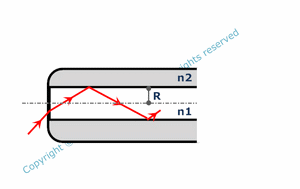Fiber optics are components guiding light. They have a cylindrical shape. They are made of a cylindrical core surrounded by a concentric cladding. The refraction index of the core ( n1 ) is larger than the refraction index of the cladding ( n2 ). In this way, internal total reflexion at the core/cladding interface occurs for incident rays with an angle of incidence smaller than a limit value ( angle of incidence being the angle between the incident ray and the fiber optic axis ). These rays are propagating in the core : they are guided in the fiber optic. However, total reflexion is not sufficient to explain light wave guiding in fiber optics.It is necessary to consider light as an electromagnetic wave. Electromagnetic equations solved considering boundaries conditions at the core/cladding interface show that only some specific waves can propagate. They are called the fiber optic modes. They have a symmetry of revolution around the fiber axis. Their intensity distribution generally contains minima and maxima. Among all possible modes, one specific mode ( the fundamental mode ) has no minima.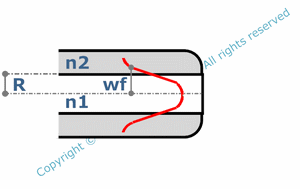For step index fiber optics ( n1 and n2 are constant ), the fundamental mode is currently modelized with a gaussian intensity distribution. Most of its power is propagating in the core. A small power amount is propagating in the cladding ( by evanescent waves ). Generally, the radius Wf of the fundamental mode ( Wf corresponding to the distance to the fiber axis for which the intensity is Imax/e2, Imax being the maximum intensity - like for laser gaussian mode ) is a little bit larger than the core radius ( R ). Wf depends on the core index ( n1 ), the cladding index ( n2 ), the wavelength ( lam ) and the core radius ( R ).

There are two different kinds of fiber optics.

Unlike multimode fiber optics, only the fundamental mode can propagate in a single mode fiber optic. A fiber optic is not intrinsically singlemode or multimode. It depends on the wavelength of the propagating wave. A fiber optic is single mode only for wavelengths larger than its cut off wavelength ( lamc ). The normalized frequency ( V ) of a fiber optic is a useful parameter for calculating lamc. It depends on n1, n2, R and the wavelength ( lam ). The fiber optic is single mode for V < 2.405. V is also practical to calculate the number of modes ( N ) able to propagate in a highly multimode fiber optic.

An important parameter is the numerical aperture ( NA ). It characterizes the maximum angle of incidence for light rays enterring in a fiber optic. NA calculation is different for singlemode and multimode fiber optics. For singlemode fibers, NA is a function of mode radius ( Wf ) and wavelength ( lam ). For multimode fibers, NA depends on n1 and n2.

Transmission

Fiber optics modify certain parameters of the light waves which propagate. In particular, fiber optics attenuate wave intensity. Transmission ( ratio between output and input power ) is a function of fiber length ( L ) and fiber attenuation factor A ( A being the attenuation by 1 km long fiber, expressed in dB/km ).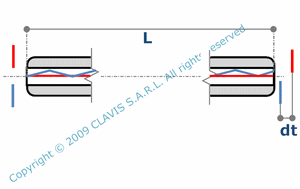In a multimode fiber, modes have different speeds. Speed decreases when mode order increases. Consequently, a fiber optic enlarges the duration of a pulse and limitates the bandwidth of propagating signals. In a step index multimode fiber, modal dispersion dt ( pulse duration spread ) depends on core index ( n1 ), cladding index ( n2 ) and fiber length ( L ). Multimode gradded index fibers have been developed to limitate modal dispersion. Their core index decreases when the distance to the fiber axis increases. A schematic explanation is given by geometric optic. Light rays having a larger angle with axis ( corresponding to higher order modes ) have a longer geometrical path in the fiber compensated by a larger speed ( because they propagate in lower index medium ).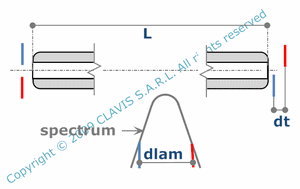Core and cladding refraction indexes always depend on wavelength. This means that waves propagating in a fiber at different wavelengths have different speeds. Real waves are never totally monochromatic : they have a certain spectrum width. Consequently their pulse duration is enlarged by the fiber optic. Chromatic dispersion ( pulse duration spread caused by indexes dispersion for a 1 nm spectrum width ) is mainly limitating the bandwidth in single mode fibers and is a function of spectrum width ( dlam ), fiber optic length ( L ) and fiber optic chromatic dispersion factor ( M ). M is the chromatic spread of a pulse with a 1 nm linewidth in a 1 km long fiber optic.

Fiber coupling

Fiber coupling is injecting light into a fiber optic. Basically, for a maximum efficiency, the beam size at the fiber input has to be the same or smaller than the core size and the beam numerical aperture has to be close to the same or smaller than the fiber numerical aperture. Practically, core diameters and numerical apertures are larger for multimode fibers than for single mode fibers. Consequently, it may be possible to inject non negligeable ratio of light coming from a reasonably large light source ( as LEDs or other non coherent light source ) in a multimode fiber. However it depends on the fiber and particulary on its numerical aperture and its core diameter which can go from a few tens of microns to a few millimeters. Coupling is generally much more tricky for single mode fibers in which only beams emitted by lasers can be efficiently injected. For classical single mode fiber optics, the best coupling ratio is obtained with gaussian beams.

Pages dedicated to he calculation of the coupling efficiency in single mode fibers ( SM fibers) consider the fiber optic mode as gaussian. Approached formulaes are used when misalignments occur and are valid only for small misalignments values.

Injecting a gaussian beam in a single mode fiber

As previously evocated, singlemode fibers request spatially coherent light from a laser or a laser diode. Ideally, the injected beam should be gaussian in order to fit with the fiber mode ( when considering the fiber mode having a gaussian intensity distribution ). The largest coupling ratio ( ratio between the input power and the power effectively propagating in the fiber ) is achieved when the beam and the fiber have the same axis, when the beam waist is located at the fiber entrance and is coinciding with the fiber mode ( waist and fiber mode have the same radius ). Depending on the initial beam, the coupling can be direct or can request some beam shaping. For example, optimal coupling can be obtained by injecting the light from a single mode fiber directly into a contiguous single mode fiber having the same core/cladding indexes and the same core radius.

On the other side, the diameter of a laser gaussian beam is too large to be directly injected in a single mode fiber. A lens is then requested to "move" the waist into the fiber entrance and adapt its radius to the fiber mode. In other situations an optical system made of two lenses may adapt a gaussian beam by collimating and then focusing it into the singlemode fiber.Whatever the solution chosen, the coupling efficiency ( C ) decreases when the waist radius ( Wo ) of the injected beam moves away from the fiber mode radius ( Wf ), but also when the beam axis defocus ( dz ) ( with fiber plane entrance as a reference ), the lateral shift ( dy ) or the tilt ( i ) ( with the fiber axis as a reference ) increase.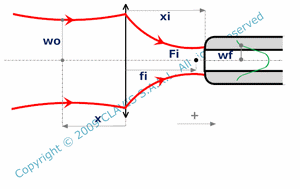The optimal lens for injecting a gaussian beam with a given waist radius Wo in a single mode fiber with a given mode radius Wf can be calculated. its effective focal length fi is a function of the wavelength ( lam ) and the distance ( x ) from the lens to the waist of the incident beam.

Injecting an elliptical beam in a single mode fiber

As evocated previously, injected beam should be ideally gaussian. Laser diodes beam don't have a symmetry of revolution. They are elliptical with different intensity distributions in the vertical and horizontal planes ( vOz and hOz ). Laser diodes with a single transverse mode, are assumed to have gaussian intensity distributions in the vertical and horizontal planes with different waists witdth. Beam emitted by other kinds of laser may also be elliptical, for example after some harmonic generation or other beam processing. In this paragraph, only elliptical beams with gaussian distributions are considered.Whatever the optical system used for coupling, an elliptical beam never fits exactly with a single mode fiber optic. Normally, the best coupling is achieved for vertical and horizontal waist in the entrance plane of the single mode fiber. Coupling ratio ( C ) depends then on the width ( Wv ) of the waist in the vertical plane, the width ( Wh ) of the waist in the horizontal plane and the fiber mode radius ( Wf ).

For a given elliptical beam with a "vertical" width ( Wv ) and an "horizontal" width ( Wh ) of the waist , it is possible to calculate the mode fiber radius ( Wf ) giving the best coupling efficiency. Wf can be calculated with Wv and the beam ellipticity ( el with el = Wh / Wv ).

As for gaussian beams, it is possible to use different ways for injecting an elliptical beam into a single mode fiber. In the general case, when using an optical system, conjugated "horizontal" and "vertical" beam waists have different positions. In the corresponding calculations, it is assumed that without any defocus, the fiber entrance is in the plane of the vertical waist of the conjugated beam. This is not necessary the optimal position.In the particular case where injection in the fiber is made using an afocal system, incident beam waists being in the front focal plane of the first lens, conjugated beam waists are both in the back focal plane of the second lens. The maximum coupling efficiency is then achieved for fiber entrance in the back focal plane of the second lens.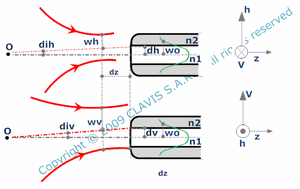The coupling ratio ( C ) changes as soon as the injected elliptical beam focus changes ( defocus dz ) or when beam axis is laterally shifted ( dh for horizontal shift and dv for vertical shift ) or tilted ( dih for tilt around vertical axis and div for tilt around horizontal axis ).

References

"Cours d'optique guidée" - Institut d'optique - 1986 - author : Serge Huart.

"Cours propagation dans les fibres optiques" - Ecole Nationale Supérieure des Télécommunications - 1988 - author : M.Monerie.

"Understanding fiber optics" - 2006 - author : Jeff Hecht.

"Cours les fibres optiques : supplément d'électromagnétisme appliqué" - Université Laval, Canada - author : Pierre-André Bélanger.

"Cours fibre optique et applications" - Institut Universitaire de Technologie Paul Cézanne - 2010 - author : Gilles Passedat.